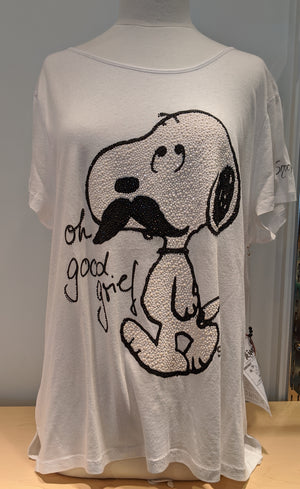Princess Goes Hollywood

# Good Grief Tee

Regular price \$135 Unit price  per

Shipping calculated at checkout.
• 50% cotton 50% modal
• Machine wash cold
• Hang to dry
• Fits true to size
• Current mood

Size Guide

• 34 = 4 = XS
• 36 = 6 = S
• 38 = 8 = M
• 40 = 10 = M
• 42 = 12 = L
• 44 = 14 = L
• 46 = 16 = XL